Problem

# Find vC(t) for t > 0 in the circuit shown in Fig. E7.1.Figure E7.1

Find vC(t) for t > 0 in the circuit shown in Fig. E7.1.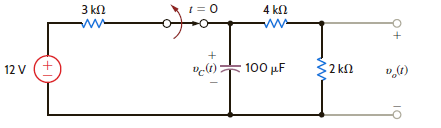Figure E7.1

#### Step-by-Step Solution

Solution 1

Refer to the Figure E7.1 in the text book.

For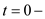, the capacitor is fully charged and conducts no current since the capacitor acts like an open circuit to dc.

Draw the following circuit diagram for.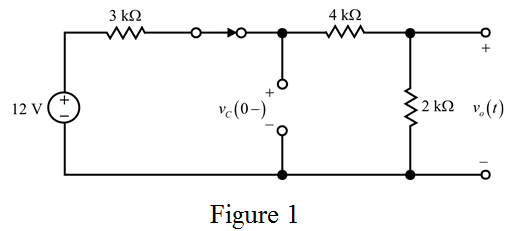Determine the initial voltage across the capacitor using voltage division.

\begin{aligned} v_{C}\left(0^{-}\right) &=12\left(\frac{4 \mathrm{k} \Omega+2 \mathrm{k} \Omega}{3 \mathrm{k} \Omega+4 \mathrm{k} \Omega+2 \mathrm{k} \Omega}\right) \\ &=12\left(\frac{6 \mathrm{k} \Omega}{9 \mathrm{k} \Omega}\right) \\ &=12\left(\frac{2}{3}\right) \\ &=8 \mathrm{~V} \end{aligned}

Therefore, the initial voltage, $$v_{C}(0-)$$ across the capacitor is $$8 \mathrm{~V}$$.

Since the voltage across the capacitor cannot change instantaneously, the voltage across the capacitor $$t>0$$ is $$8 \mathrm{~V}$$.

Therefore, $$v_{C}(0+)=8 \mathrm{~V}$$.

At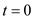the switch is opens. For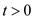, the circuit is shown in Figure 2.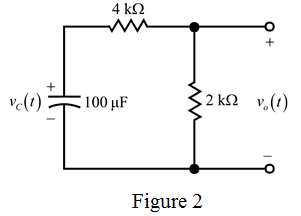Apply Kirchhoff's current law to the circuit shown in Figure $$2 .$$

$$(100 \mu) \frac{d v_{C}(t)}{d t}+\frac{v_{C}(t)}{6 \mathrm{k}}=0$$

$$\frac{d v_{C}(t)}{d t}+\frac{v_{C}(t)}{(6 \mathrm{k})(100 \mu)}=0$$

$$\frac{d v_{c}(t)}{d t}+\frac{v_{c}(t)}{0.6}=0 \ldots \ldots .(1)$$

The equation (1) is in the form of $$\frac{d x_{c}(t)}{d t}+a x_{c}(t)=0$$.

Where,

$$x_{C}(t)=v_{C}(t)$$

$$a=\frac{1}{0.6}$$

The form of the solution to this homogeneous equation is,

$$v_{C}(t)=K_{2} e^{-a t} \mathrm{~V}$$

$$v_{C}(t)=K_{2} e^{-\frac{1}{0.6} \ldots \ldots(2)}$$

Substitute $$t=0$$ in equation (2). $$v_{C}(0)=K_{2} e^{-\frac{0}{0.6}}$$

$$8=K_{2}(1)$$

$$K_{2}=8$$

Substitute $$K_{2}=8$$ in equation (2).

$$v_{C}(t)=8 e^{\frac{1}{0.6}} \mathrm{~V}$$

Therefore, the voltage across the capacitor, $$v_{C}(t)$$ for $$t>0$$ is $$8 e^{-\frac{1}{0.6}} \mathrm{~V}$$.